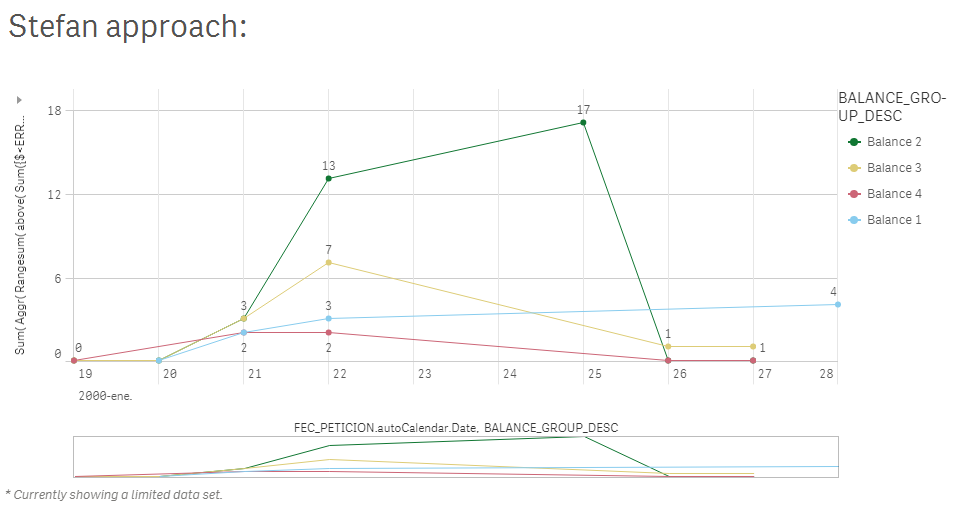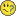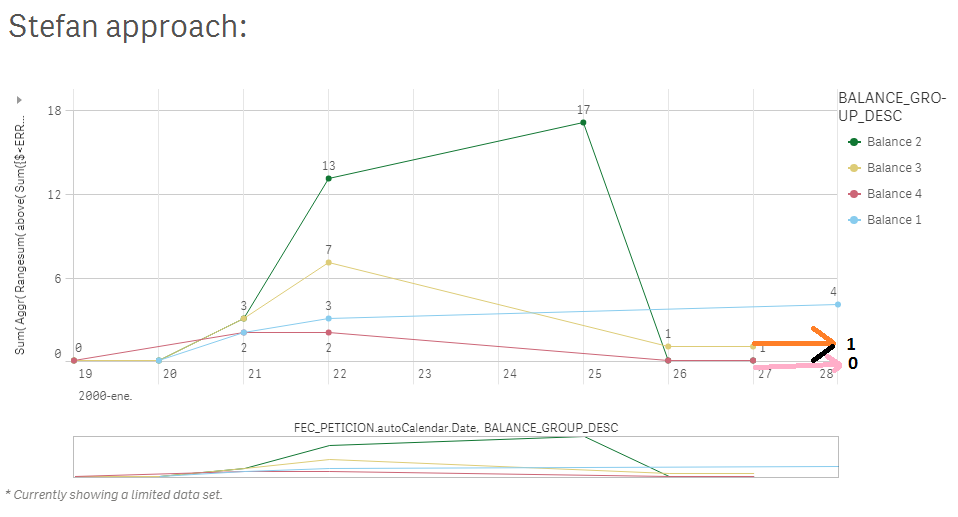# Qlik Sense App Development

Discussion board where members can learn more about Qlik Sense App Development and Usage.

Highlighted
New Contributor III

## Ignore one dimension in above function

Hi,

I'd like to plot a chart with a cumulative sum over time, and split it into groups (one cumulative sum per group)

I tried a line chart with two dimensions FEC_PETICION (date) and BALANCE_GROUP_DESC (group description string)
And the next measure:

Rangesum(
above(Sum({\$<ERROR={0}>}[BALANCE_FACTOR]),
0,
vMaxDays
)
)

It's not working because the BALANCE_GROUP_DESC is interfering the cumulative sum.

I'd tried the next, but QlikSense doesn't allow exceptions to TOTAL clause for above function ¿?

Rangesum(
above(TOTAL<FEC_PETICION> Sum({\$<ERROR={0}>}[BALANCE_FACTOR]),
0,
vMaxDays
)
)

How could I ignore the date FEC_PETICION for cumulative sum, but use it to draw separately each cumulative sum into groups?

A sample application is attached.

TIA,

Virilo

Tags (4)
1 Solution

Accepted SolutionsMVP

## Re: Ignore one dimension in above function

May be your date isn't sorted correctly in the script... and you need this

Sum(

Aggr(

Rangesum(

above( Sum({\$<ERROR={0}>}[BALANCE_FACTOR]),

0,

vMaxDays

)

)

, BALANCE_GROUP_DESC , (FEC_PETICION.autoCalendar.Date, (NUMERIC))

)

)5 RepliesMVP

## Re: Ignore one dimension in above function

Try something like

Sum( Aggr(

Rangesum(

above( Sum({\$<ERROR={0}>}[BALANCE_FACTOR]),

0,

vMaxDays

)

)

, BALANCE_GROUP_DESC , FEC_PETICION

))

Note the swapped dimension order in the Aggr() dimension list.

New Contributor III

## Re: Ignore one dimension in above function

Hi Stefan,

Thanks a lot.  I read https://help.qlik.com/en-US/sense/June2018/Subsystems/Hub/Content/ChartFunctions/aggr.htm and tried your solution without successI have attached a sample application if it helps.

Best regards,

ViriloMVP

## Re: Ignore one dimension in above function

May be your date isn't sorted correctly in the script... and you need this

Sum(

Aggr(

Rangesum(

above( Sum({\$<ERROR={0}>}[BALANCE_FACTOR]),

0,

vMaxDays

)

)

, BALANCE_GROUP_DESC , (FEC_PETICION.autoCalendar.Date, (NUMERIC))

)

)New Contributor III

## Re: Ignore one dimension in above function

Thanks a lot swuehl, stalwar1,

It is working!

Is it there any way to extend all the data points to the right?

I'd like people to see that at the most recent day (28-jan), the balance is:

Balance1: 4 pending messages
Balance2: 0 pending messages
Balance3: 1 pending message
Balance4: 0 pending messagesBest regards,

ViriloMVP

## Re: Ignore one dimension in above function

May be you will need to Generating Missing Data In QlikView‌ to get this done...